# 根据数据驱动技术进行配对交易

Author: , Created: 2019-08-21 13:50:23, Updated: 2019-08-23 10:59:28

# 解释概念：两个假设的投资标的

• 在发明者量化平台搭建我们的研究环境

``````import numpy as np
import pandas as pd

import statsmodels
from statsmodels.tsa.stattools import coint
# just set the seed for the random number generator
np.random.seed(107)

import matplotlib.pyplot as plt
``````

``````# Generate daily returns
Xreturns = np.random.normal(0, 1, 100)
# sum them and shift all the prices up
X = pd.Series(np.cumsum(
Xreturns), name='X')
+ 50
X.plot(figsize=(15,7))
plt.show()
``````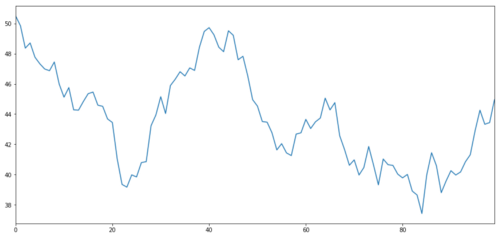``````noise = np.random.normal(0, 1, 100)
Y = X + 5 + noise
Y.name = 'Y'
pd.concat([X, Y], axis=1).plot(figsize=(15,7))
plt.show()
``````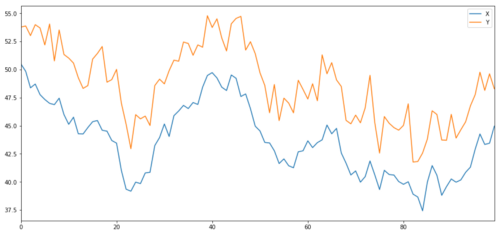## 协整

Y = ⍺ X + e

``````(Y/X).plot(figsize=(15,7))
plt.axhline((Y/X).mean(), color='red', linestyle='--')
plt.xlabel('Time')
plt.legend(['Price Ratio', 'Mean'])
plt.show()
``````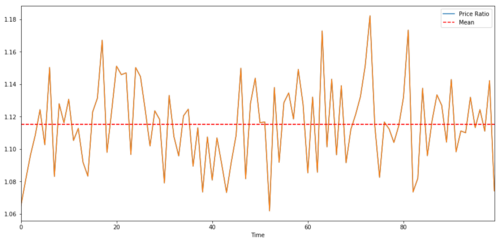## 协整测试

``````# compute the p-value of the cointegration test
# will inform us as to whether the ratio between the 2 timeseries is stationary
# around its mean
score, pvalue, _ = coint(X,Y)
print pvalue
``````

## 注意：相关性与协整性

``````X.corr(Y)
``````

``````ret1 = np.random.normal(1, 1, 100)
ret2 = np.random.normal(2, 1, 100)

s1 = pd.Series( np.cumsum(ret1), name='X')
s2 = pd.Series( np.cumsum(ret2), name='Y')

pd.concat([s1, s2], axis=1 ).plot(figsize=(15,7))
plt.show()
print 'Correlation: ' + str(X_diverging.corr(Y_diverging))
score, pvalue, _ = coint(X_diverging,Y_diverging)
print 'Cointegration test p-value: ' + str(pvalue)
``````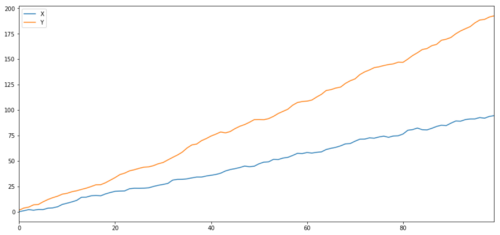``````Y2 = pd.Series(np.random.normal(0, 1, 800), name='Y2') + 20
Y3 = Y2.copy()
``````
``````Y3[0:100] = 30
Y3[100:200] = 10
Y3[200:300] = 30
Y3[300:400] = 10
Y3[400:500] = 30
Y3[500:600] = 10
Y3[600:700] = 30
Y3[700:800] = 10
Y2.plot(figsize=(15,7))
Y3.plot()
plt.ylim([0, 40])
plt.show()
# correlation is nearly zero
print 'Correlation: ' + str(Y2.corr(Y3))
score, pvalue, _ = coint(Y2,Y3)
print 'Cointegration test p-value: ' + str(pvalue)
``````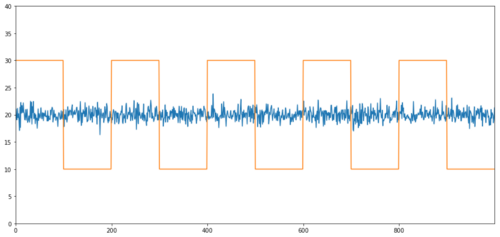# 如何进行配对交易？

• 做多比率:这是当比率⍺很小并且我们预计它变大时。在上面的例子中，我们通过做多Y并做空X来开仓。

• 做空比率:这是当比率⍺很大并且我们期望它变小时。在上面的例子中，我们通过做空Y和做多X来开仓。

# 使用数据查找行为类似的交易标的

``````def find_cointegrated_pairs(data):
n = data.shape
score_matrix = np.zeros((n, n))
pvalue_matrix = np.ones((n, n))
keys = data.keys()
pairs = []
for i in range(n):
for j in range(i+1, n):
S1 = data[keys[i]]
S2 = data[keys[j]]
result = coint(S1, S2)
score = result
pvalue = result
score_matrix[i, j] = score
pvalue_matrix[i, j] = pvalue
if pvalue < 0.02:
pairs.append((keys[i], keys[j]))
return score_matrix, pvalue_matrix, pairs
``````

``````from backtester.dataSource.yahoo_data_source import YahooStockDataSource
from datetime import datetime
startDateStr = '2007/12/01'
endDateStr = '2017/12/01'
cachedFolderName = 'yahooData/'
'HPQ','JNPR','AMD','IBM']
ds = YahooStockDataSource(cachedFolderName=cachedFolderName,
dataSetId=dataSetId,
instrumentIds=instrumentIds,
startDateStr=startDateStr,
endDateStr=endDateStr,
event='history')
``````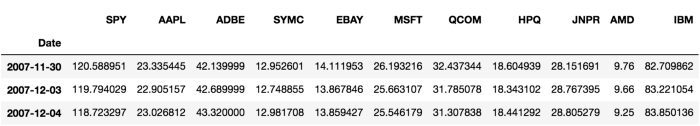``````# Heatmap to show the p-values of the cointegration test
# between each pair of stocks
scores, pvalues, pairs = find_cointegrated_pairs(data)
import seaborn
m = [0,0.2,0.4,0.6,0.8,1]
seaborn.heatmap(pvalues, xticklabels=instrumentIds,
yticklabels=instrumentIds, cmap=’RdYlGn_r’,
plt.show()
print pairs
``````
``````[('ADBE', 'MSFT')]
````````````S1 = data['ADBE']
S2 = data['MSFT']
score, pvalue, _ = coint(S1, S2)
print(pvalue)
ratios = S1 / S2
ratios.plot()
plt.axhline(ratios.mean())
plt.legend([' Ratio'])
plt.show()
``````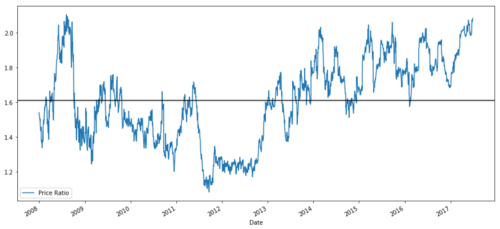Z Score (Value) = (Value — Mean) / Standard Deviation

## 警告

``````def zscore(series):
return (series - series.mean()) / np.std(series)
``````
``````zscore(ratios).plot()
plt.axhline(zscore(ratios).mean())
plt.axhline(1.0, color=’red’)
plt.axhline(-1.0, color=’green’)
plt.show()
``````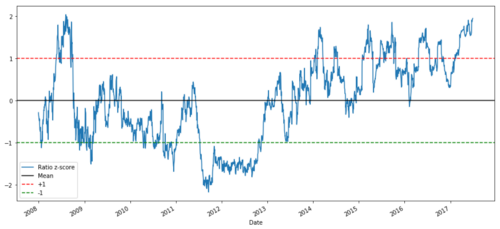• 收集可靠的数据和清理数据

• 从数据创建功能以识别交易信号/逻辑

• 功能可以是移动平均值或价格数据，相关性或更复杂信号的比率 - 将这些组合起来创建新功能

• 使用这些功能生成交易信号，即哪些信号是买入，卖出或空仓观望

## 第一步：设置你的问题

Y = Ratio is buy (1) or sell (-1)

Y(t)= Sign(Ratio(t+1) — Ratio(t))

## 第三步：拆分数据

• Training 7 years ~ 70%

• Test ~ 3 years 30%

``````ratios = data['ADBE'] / data['MSFT']
print(len(ratios))
train = ratios[:1762]
test = ratios[1762:]
``````

## 第四步：特征工程

• 60天移动平均比率：滚动平均值的测量

• 5天移动平均比率：平均值的当前值的测量

• 60天标准差

• z分数：（5d MA - 60d MA）/ 60d SD

``````ratios_mavg5 = train.rolling(window=5,
center=False).mean()
ratios_mavg60 = train.rolling(window=60,
center=False).mean()
std_60 = train.rolling(window=60,
center=False).std()
zscore_60_5 = (ratios_mavg5 - ratios_mavg60)/std_60
plt.figure(figsize=(15,7))
plt.plot(train.index, train.values)
plt.plot(ratios_mavg5.index, ratios_mavg5.values)
plt.plot(ratios_mavg60.index, ratios_mavg60.values)
plt.legend(['Ratio','5d Ratio MA', '60d Ratio MA'])
plt.ylabel('Ratio')
plt.show()
``````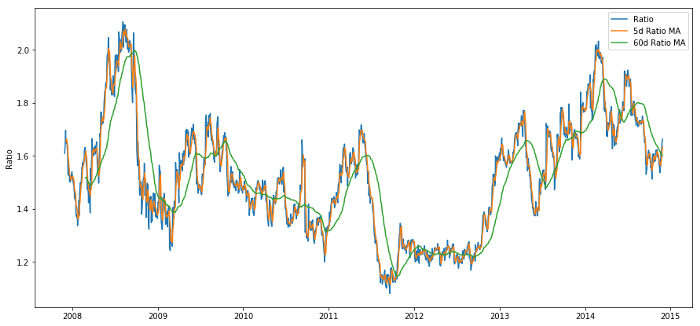60d和5d MA的价格比率

``````plt.figure(figsize=(15,7))
zscore_60_5.plot()
plt.axhline(0, color='black')
plt.axhline(1.0, color='red', linestyle='--')
plt.axhline(-1.0, color='green', linestyle='--')
plt.legend(['Rolling Ratio z-Score', 'Mean', '+1', '-1'])
plt.show()
``````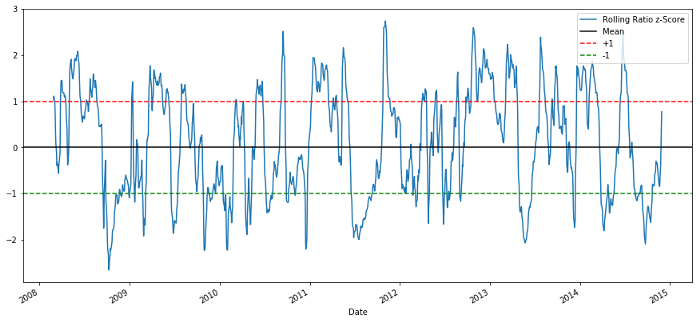60-5 Z分数价格比例

## 第五步：模型选择

• 当z低于-1.0，比率就是买（1），因为我们预计z会回归到0，因此比率增加

• 当z高于1.0时，比率是卖出（-1），因为我们预计z会回归到0，因此比率降低

## 第六步：训练，验证和优化

``````# Plot the ratios and buy and sell signals from z score
plt.figure(figsize=(15,7))
train[60:].plot()
sell = train.copy()
sell[zscore_60_5<1] = 0
sell[60:].plot(color=’r’, linestyle=’None’, marker=’^’)
x1,x2,y1,y2 = plt.axis()
plt.axis((x1,x2,ratios.min(),ratios.max()))
plt.show()
``````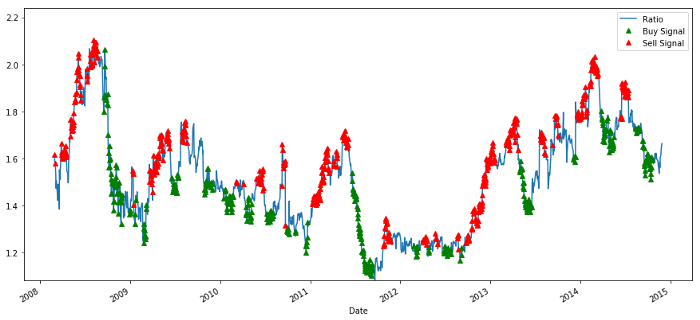``````# Plot the prices and buy and sell signals from z score
plt.figure(figsize=(18,9))
S2 = data['MSFT'].iloc[:1762]
S1[60:].plot(color='b')
S2[60:].plot(color='c')
sellR = 0*S1.copy()
# When selling the ratio, sell S1 and buy S2
sellR[sell!=0] = S1[sell!=0]
sellR[60:].plot(color='r', linestyle='None', marker='^')
x1,x2,y1,y2 = plt.axis()
plt.axis((x1,x2,min(S1.min(),S2.min()),max(S1.max(),S2.max())))
plt.show()
``````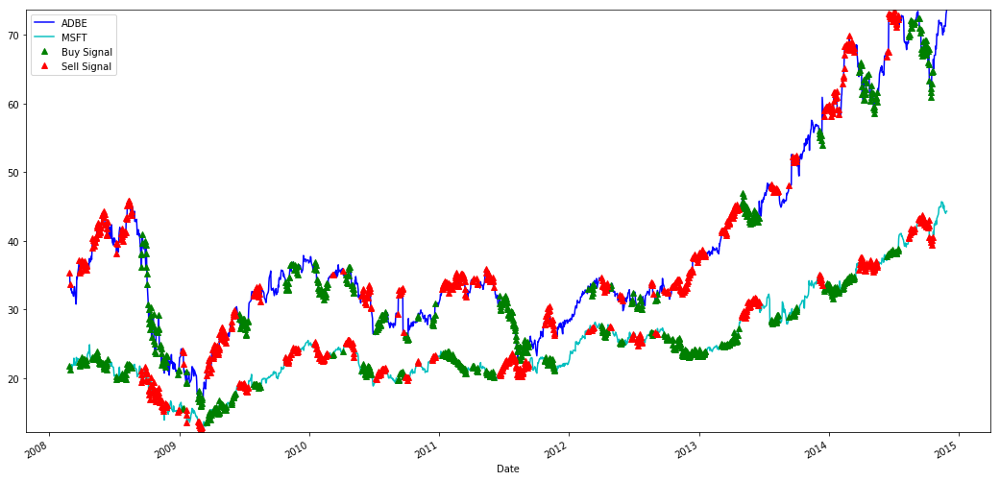``````# Trade using a simple strategy

# If window length is 0, algorithm doesn't make sense, so exit
if (window1 == 0) or (window2 == 0):
return 0

# Compute rolling mean and rolling standard deviation
ratios = S1/S2
ma1 = ratios.rolling(window=window1,
center=False).mean()
ma2 = ratios.rolling(window=window2,
center=False).mean()
std = ratios.rolling(window=window2,
center=False).std()
zscore = (ma1 - ma2)/std

money = 0
countS1 = 0
countS2 = 0
for i in range(len(ratios)):
# Sell short if the z-score is > 1
if zscore[i] > 1:
money += S1[i] - S2[i] * ratios[i]
countS1 -= 1
countS2 += ratios[i]
print('Selling Ratio %s %s %s %s'%(money, ratios[i], countS1,countS2))
# Buy long if the z-score is < 1
elif zscore[i] < -1:
money -= S1[i] - S2[i] * ratios[i]
countS1 += 1
countS2 -= ratios[i]
print('Buying Ratio %s %s %s %s'%(money,ratios[i], countS1,countS2))
# Clear positions if the z-score between -.5 and .5
elif abs(zscore[i]) < 0.75:
money += S1[i] * countS1 + S2[i] * countS2
countS1 = 0
countS2 = 0
print('Exit pos %s %s %s %s'%(money,ratios[i], countS1,countS2))

return money
``````

## 第七步：对测试数据进行回测

``````trade(data[‘ADBE’].iloc[1762:], data[‘MSFT’].iloc[1762:], 60, 5)
``````

# 避免过度拟合

``````# Find the window length 0-254
# that gives the highest returns using this strategy
data['MSFT'].iloc[:1762], l, 5)
for l in range(255)]
best_length = np.argmax(length_scores)
print ('Best window length:', best_length)
``````
``````('Best window length:', 40)
``````

``````# Find the returns for test data
# using what we think is the best window length
data['MSFT'].iloc[1762:],l,5)
for l in range(255)]
print (best_length, 'day window:', length_scores2[best_length])
# Find the best window length based on this dataset,
# and the returns using this window length
best_length2 = np.argmax(length_scores2)
print (best_length2, 'day window:', length_scores2[best_length2])
``````
``````(40, 'day window:', 1252233.1395)
(15, 'day window:', 1449116.4522)
``````

``````plt.figure(figsize=(15,7))
plt.plot(length_scores)
plt.plot(length_scores2)
plt.xlabel('Window length')
plt.ylabel('Score')
plt.legend(['Training', 'Test'])
plt.show()
``````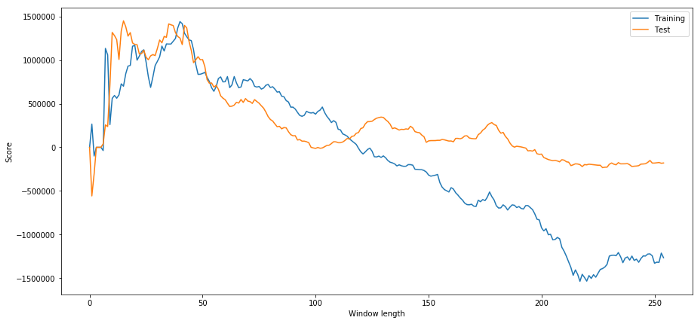# 下一步

• 赫斯特指数

• 从Ornstein-Uhlenbeck过程推断的均值回归的半衰期

• 卡尔曼滤波器

More

bk_fund 没有找到这个包

bk_fund 哪里可以安装backtester.dataSource.yahoo_data_source